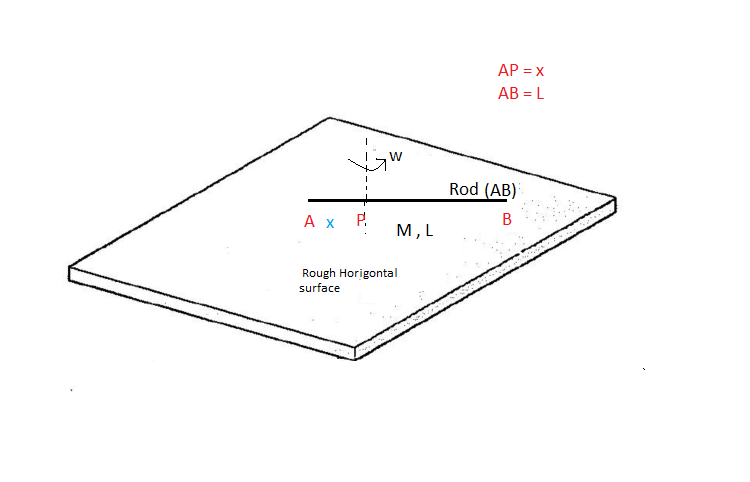# Variable Friction on horizontal Rod !!Let a uniform rod of mass $M$ and length $L$ is rotating with angular velocity $\omega$ about an point $P$ which is at the position of $x$ from one end of rod . At time zero, the rod is placed gently on a rough, level surface having coefficient of friction $\mu$.

If the total time taken by the rod to stop completely $T$, then for different values of position $x$, the time taken by the rod in various experiments are different.

Suppose the maximum possible time taken for the rod to slow is ${ T }_{ max }$ and the minimum possible time is ${ T }_{ min }$, find the value of :

$100\quad \times \quad \cfrac { { T }_{ min } }{ { T }_{ max } }$.

Details

• $L$ = 10 m
• $M$ = 10 kg
• $\mu$ = 0.5
• $g$ = 10 m/s^2
• $\omega$ = 10 rad/s